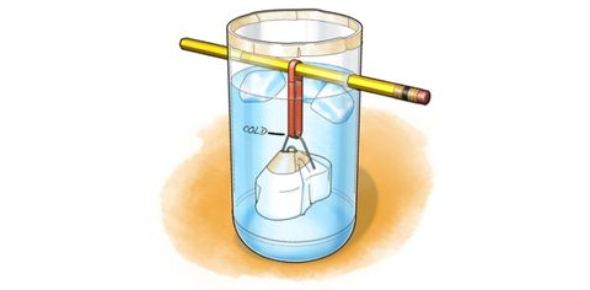# Thermal Physics Exam Trivia Quiz!

8 Questions | Attempts: 241Settings.

• 1.
A train begins a journey from a station and travels 60km in a time of 20 minutes. What is the average speed of the train?
• A.

3.0 m/s

• B.

5.0 m/s

• C.

50 m/s

• D.

60 m/s

• 2.
Two runners take part in a race. The graph below shows how the speed of each runner changes with time. What does the graph show about the runners at time t?
• A.

Both runners are moving at the same speed.

• B.

Runner 1 has zero acceleration.

• C.

Runner 1 is overtaking runner 2.

• D.

Runner 2 is slowing down.

• 3.
A large stone is dropped from a bridge into a river. Air resistance can be ignored. Which row describes the acceleration and the speed of the stone as it falls?
• A.

Constant acceleration of stone

• B.

Increasing acceleration of stone

• C.

Constant speed of stone

• D.

Increasing speed of stone

• 4.
An object moves at a constant speed for some time, then begins to accelerate. Which distance-time graph shows this motion?
• A.

A

• B.

B

• C.

C

• D.

D

• 5.
The diagram shows the speed-time graph for a car. Which area represents the distance traveled while the car is accelerating?
• A.

X

• B.

X +Y

• C.

Y

• D.

Y-X

• 6.
Two distance-time graphs and two speed-time graphs are shown. Which graph represents an object that is at rest?
• A.

A

• B.

B

• C.

C

• D.

D

• 7.
A car travels 100km. The journey takes two hours. The highest speed of the car is 80km/ h, and the lowest speed is 40km/ h. What is the average speed for the journey?
• A.

40km/h

• B.

50km/h

• C.

60km/h

• D.

120km/h

• 8.
A car travels along the route PQRST in 30 minutes. What is the average speed of the car?
• A.

10km/hr

• B.

20km/hr

• C.

30km/hr

• D.

60km/hr

## Related TopicsBack to top
×

Wait!
Here's an interesting quiz for you.